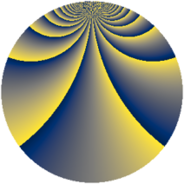# Properties

 Label 1104.2.boLevel $1104$ Weight $2$ Character orbit 1104.bo Rep. character $\chi_{1104}(5,\cdot)$ Character field $\Q(\zeta_{44})$ Dimension $3760$ Sturm bound $384$

# Related objects

## Defining parameters

 Level: $$N$$ $$=$$ $$1104 = 2^{4} \cdot 3 \cdot 23$$ Weight: $$k$$ $$=$$ $$2$$ Character orbit: $$[\chi]$$ $$=$$ 1104.bo (of order $$44$$ and degree $$20$$) Character conductor: $$\operatorname{cond}(\chi)$$ $$=$$ $$1104$$ Character field: $$\Q(\zeta_{44})$$ Sturm bound: $$384$$

## Dimensions

The following table gives the dimensions of various subspaces of $$M_{2}(1104, [\chi])$$.

Total New Old
Modular forms 3920 3920 0
Cusp forms 3760 3760 0
Eisenstein series 160 160 0

## Trace form

 $$3760q - 18q^{3} - 36q^{4} - 24q^{6} + O(q^{10})$$ $$3760q - 18q^{3} - 36q^{4} - 24q^{6} - 44q^{10} - 24q^{12} - 36q^{13} - 44q^{15} - 76q^{16} - 10q^{18} - 44q^{19} - 22q^{21} - 40q^{24} - 6q^{27} - 44q^{28} - 22q^{30} - 72q^{31} - 44q^{33} - 88q^{34} - 28q^{36} - 44q^{37} - 44q^{40} - 22q^{42} - 44q^{43} + 100q^{46} - 30q^{48} - 400q^{49} - 22q^{51} - 4q^{52} - 18q^{54} - 184q^{58} - 22q^{60} - 44q^{61} - 44q^{63} - 72q^{64} - 22q^{66} - 44q^{67} - 14q^{69} - 24q^{70} + 70q^{72} - 10q^{75} - 44q^{76} - 56q^{78} - 88q^{79} - 36q^{81} - 24q^{82} - 22q^{84} + 36q^{85} - 44q^{88} - 22q^{90} - 268q^{93} + 36q^{94} - 86q^{96} - 88q^{97} - 22q^{99} + O(q^{100})$$

## Decomposition of $$S_{2}^{\mathrm{new}}(1104, [\chi])$$ into newform subspaces

The newforms in this space have not yet been added to the LMFDB.